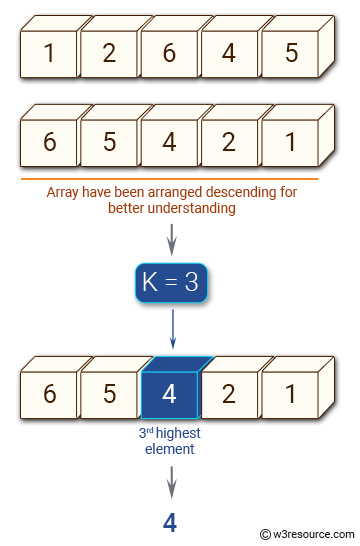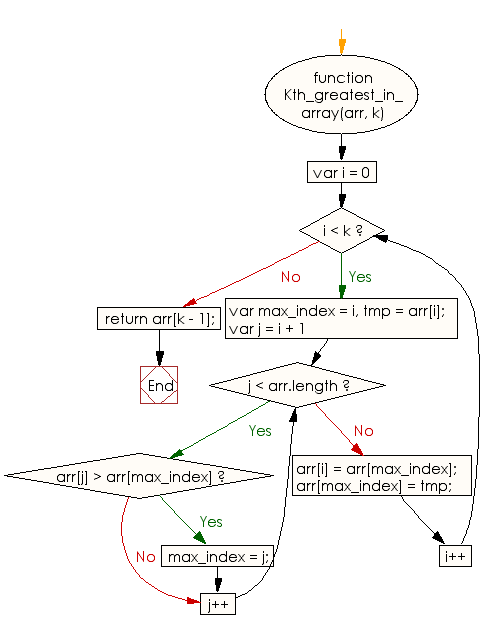# JavaScript: Find the kth greatest element of a given array of integers

## JavaScript Basic: Exercise-90 with Solution

Write a JavaScript program to find the kth greatest element of a given array of integers.

Pictorial Presentation:Sample Solution:

HTML Code:

``````<!DOCTYPE html>
<html>
<meta charset="utf-8">
<meta name="viewport" content="width=device-width">
<title>Find the kth greatest element of a given array of integers.</title>
<body>

</body>
</html>
```
```

JavaScript Code:

``````function Kth_greatest_in_array(arr, k) {

for (var i = 0; i < k; i++) {
var max_index = i,
tmp = arr[i];

for (var j = i + 1; j < arr.length; j++) {
if (arr[j] > arr[max_index]) {
max_index = j;
}
}

arr[i] = arr[max_index];
arr[max_index] = tmp;
}

return arr[k - 1];
}

console.log(Kth_greatest_in_array([1,2,6,4,5], 3))
console.log(Kth_greatest_in_array([-10,-25,-47,-36,0], 1))
```
```

Sample Output:

```4
0
```

Flowchart:ES6 Version:

``````function Kth_greatest_in_array(arr, k) {

for (let i = 0; i < k; i++) {
let max_index = i;
const tmp = arr[i];

for (let j = i + 1; j < arr.length; j++) {
if (arr[j] > arr[max_index]) {
max_index = j;
}
}

arr[i] = arr[max_index];
arr[max_index] = tmp;
}

return arr[k - 1];
}

console.log(Kth_greatest_in_array([1,2,6,4,5], 3))
console.log(Kth_greatest_in_array([-10,-25,-47,-36,0], 1))
``````

Live Demo:

See the Pen javascript-basic-exercise-90 by w3resource (@w3resource) on CodePen.

What is the difficulty level of this exercise?

Test your Programming skills with w3resource's quiz.

﻿

## JavaScript: Tips of the Day

Returns the sum of the powers of all the numbers from start to end (both inclusive)

Example:

```const sumPower = (end, power = 2, start = 1) =>
Array(end + 1 - start)
.fill(0)
.map((x, i) => (i + start) ** power)
.reduce((a, b) => a + b, 0);
console.log(sumPower(10)); // 385
console.log(sumPower(10, 3)); // 3025
console.log(sumPower(10, 3, 5)); // 2925
```

Output:

```385
3025
2925
```